# Finsler space, generalized

(diff) ← Older revision | Latest revision (diff) | Newer revision → (diff)

A space with an internal metric, subject to certain restrictions on the behaviour of shortest curves (that is, curves with length equal to the distance between their ends). Such spaces include-spaces (see Geodesic geometry) and, in particular, Finsler spaces (cf. Finsler geometry), so that the spaces in question can be characterized as a generalization of Finsler, and not just of Riemannian spaces. Generalized Finsler spaces differ from Finsler spaces not only in their greater generality, but also in the fact that they are defined and investigated starting from a metric, without coordinates.

A-space can be defined as a finitely compact space (that is, closed bounded sets in it are compact) with an internal metric in which shortest curves are locally uniquely extendable, that is, such that the following two conditions are satisfied.

1) Existence of an extension: Each point has a neighbourhoodsuch that for every shortest curve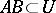there is a shortest curve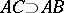,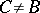.

2) Uniqueness of the extension, or "non-superposition" : Ifand, then eitheror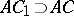. The actual existence of shortest curves is ensured by finite compactness: In a finitely compact space with an internal metric any two points can be joined by a shortest curve. The uniqueness of the extension implies the local uniqueness of the shortest curve with given end-points. Thus, a-space can be characterized as a finitely compact space in which any two points can be joined by a shortest curve that locally possesses the main properties of straight line-segments.

For spaces with an internal metric, finite compactness is equivalent to the combination of local compactness and completeness (see ).

A-space is topologically homogeneous, that is, for any two points in it there is a homeomorphism of the space onto itself that maps one of these points to the other. Moreover, this homeomorphism can be chosen to be isotopic to the identity.-spaces of dimensionare topological manifolds. A finite-dimensional-space has the property of invariance of domains, that is, if one of two homeomorphic subsets of it is open, then so is the other. It is not known (1984) whether every-space is finite-dimensional.

A setin a spacewith an internal metric is called convex if any two pointscan be joined by a shortest curveand if every such shortest curve is contained in. A spacehas the property of convexity of small spheres if every point of it has a neighbourhood such that every sphere contained in it is convex. Every-space with convex small spheres is finite-dimensional. In particular, this is true for-spaces of curvature(see Riemannian space, generalized) and for-spaces of non-positive curvature (in the sense of Busemann). The latter means that locally in every triangle consisting of shortest curves the length of a middle line of the triangle is not greater than half the corresponding side. The finite-dimensionality is also true for-spaces of curvature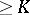(see ).

A motion of a metric space is a mapping of the space onto itself that preserves distances between points. The group of all motions of a compact-space with the compact-open topology is a Lie group (see ). This is also true in the non-compact case if the-space satisfies the condition of convexity of small spheres (see ).

A metric space is homogeneous if for any two points of the space there is a motion mapping one of the points to the other. A motionof a metric space is called a symmetry relative to a pointif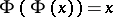for all, and ifis an isolated fixed point of. A space is called symmetric if for any point of it there is a symmetry relative to that point. Symmetric-spaces are homogeneous and satisfy the condition of convexity of small spheres. The group of all motions of a homogeneous-space is a Lie group, and the space itself is a topological manifold (see ). A symmetric-space is a Finsler space with convex indicatrices. If, in addition, there is an angle between any two shortest curves emanating from one point, then the space is a symmetric Riemannian space.

Letbe a metric space with metric, endowed with a group structure. The metricis called left-invariant if the mapping of left multiplication by an elementis a motion of the space. A right-invariant metric is defined similarly. A metric that is both left- and right-invariant is called bi-invariant. A-space that is a group with a bi-invariant metric is a symmetric Lie group (see ), and consequently a Finsler space. This is a generalization of a well-known result for Riemannian spaces.

How to Cite This Entry:
Finsler space, generalized. Encyclopedia of Mathematics. URL: http://encyclopediaofmath.org/index.php?title=Finsler_space,_generalized&oldid=14337
This article was adapted from an original article by A.D. AleksandrovV.N. Berestovskii (originator), which appeared in Encyclopedia of Mathematics - ISBN 1402006098. See original article